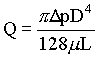### Capillary Tube Viscosity Measurements

A capillary tube 0.2 cm in diameter and 10 cm long discharge one liter of a liquid in ten minutes under a pressure difference of 5 cm mercury. Find the viscosity of the liquid using the following data:
Density of oil = 850 kg/m3, Density of mercury = 13600 kg/m3

Data:

Dia of tube(D) = 0.2 cm = 0.002 m

Length of pipe(L) = 10 cm = 0.1 m

Density of mercury = 13600 kg/m3

Density of oil(r) = 850 kg/m3

Pressure drop(Dp) = 5 cm Hg = 0.05 x 13600 x 9.812 N/m2 = 6672.16 N/m2

Flow rate(Q) = 1 litre/10 min = 0.001/(10 x 60) = 1.667 x 10-6 m3/sec

Formula:

Hagen-Poiseuille law (pressure drop for laminar flow is related to the flow parameters)Calculations:

In capillary tube viscosity measurements the flow is maintained in laminar conditions.

m = (p x 6672.16 x 0.0024)/(1.667 x 10-6 x 128 x 0.1) = = 0.01572 kg/(m.sec) = 15.72 cP

HOME